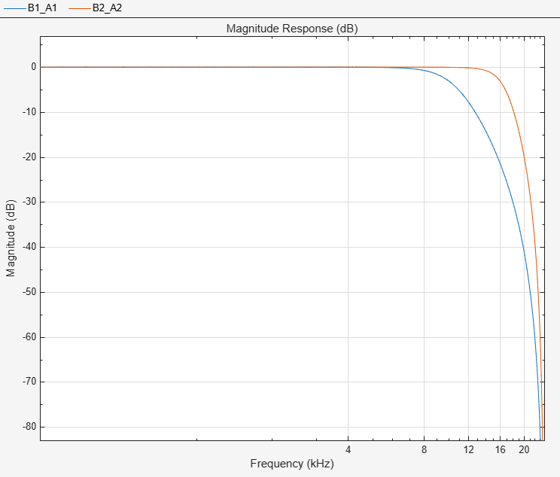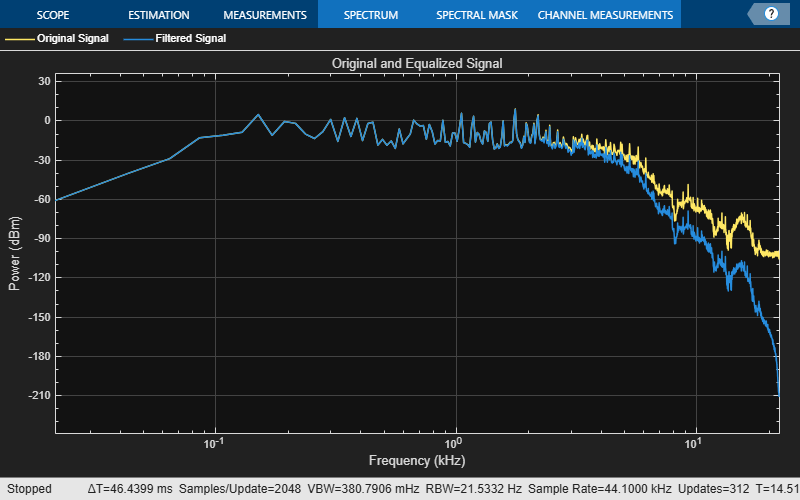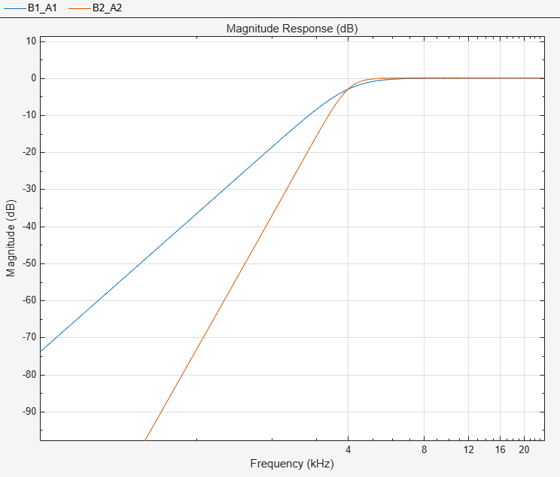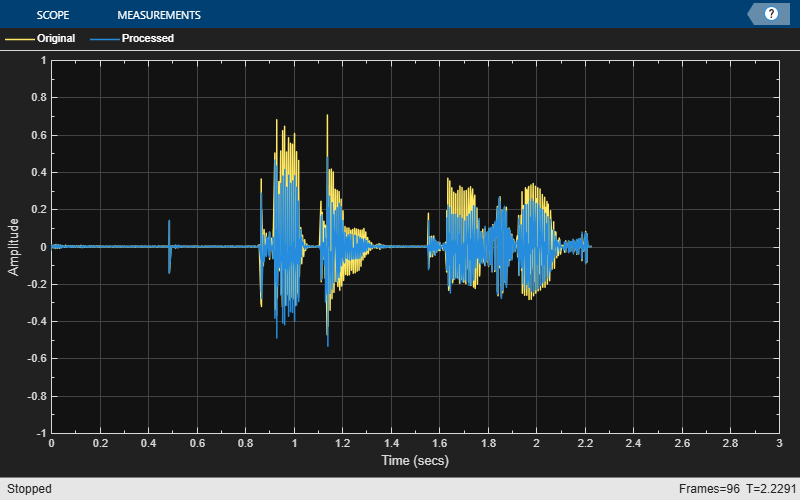# designVarSlopeFilter

Design variable slope lowpass or highpass IIR filter

## Syntax

``````[B,A] = designVarSlopeFilter(slope,Fc)``````
``````[B,A] = designVarSlopeFilter(slope,Fc,type)``````
``````[B,A] = designVarSlopeFilter(___,Name,Value)``````

## Description

example

``````[B,A] = designVarSlopeFilter(slope,Fc)``` designs a lowpass filter with the specified slope and cutoff frequency. `B` and `A` are matrices of numerator and denominator coefficients, with columns corresponding to cascaded second-order sections (SOS). ```

example

``````[B,A] = designVarSlopeFilter(slope,Fc,type)``` specifies the design type as a lowpass or highpass filter.```
``````[B,A] = designVarSlopeFilter(___,Name,Value)``` specifies options using one or more `Name,Value` pair arguments.```

## Examples

collapse all

Design two second-order section (SOS) lowpass IIR filters using `designVarSlopeFilter`.

Specify the sampling frequency, slope, and normalized cutoff frequency for two lowpass IIR filters. The sampling frequency is in Hz. The slope is in dB/octave.

```Fs = 48e3; slope = 18; Fc1 = 10e3/(Fs/2); Fc2 = 16e3/(Fs/2);```

Design the filter coefficients using the specified parameters.

```[B1,A1] = designVarSlopeFilter(slope,Fc1,"Orientation","row"); [B2,A2] = designVarSlopeFilter(slope,Fc2,"Orientation","row");```

```fvt = fvtool([B1,A1],[B2,A2],Fs=Fs,FrequencyScale="log"); legend(fvt,"Fc = 10 kHz","Fc = 16 kHz",Location="southwest")```Design a second-order section (SOS) lowpass IIR filter using `designVarSlopeFilter`. Use your lowpass filter to process an audio signal.

Create audio file reader and audio device writer objects. Use the sample rate of the reader as the sample rate of the writer.

```frameSize = 256; fileReader = dsp.AudioFileReader( ... "RockGuitar-16-44p1-stereo-72secs.wav", ... SamplesPerFrame=frameSize); sampleRate = fileReader.SampleRate; deviceWriter = audioDeviceWriter( ... SampleRate=sampleRate);```

Play the audio signal through your device.

```count = 0; while count < 2500 audio = fileReader(); deviceWriter(audio); count = count + 1; end reset(fileReader)```

Design a lowpass filter with a 12 dB/octave slope and a 0.15 normalized cutoff frequency.

```slope = 12; cutoff = 0.15; [B,A] = designVarSlopeFilter(slope,cutoff);```

Visualize your filter design. To output filter coefficients suitable for `freqz`, call `designVarSlopeFilter` again with the same design specifications but with `Orientation` set to `"row"`.

```[Bvisualize,Avisualize] = designVarSlopeFilter(slope,cutoff,Orientation="row"); [h,f] = freqz([Bvisualize Avisualize],[],sampleRate); plot(f/1000,mag2db(abs(h))) grid ylim([-75 5]) xlabel("Frequency (kHz)") ylabel("Magnitude Response (dB)")``````myFilter = dsp.BiquadFilter( ... SOSMatrixSource="Input port", ... ScaleValuesInputPort=false);```

Create a spectrum analyzer to visualize the original audio signal and the audio signal passed through your lowpass filter.

```scope = dsp.SpectrumAnalyzer( ... SampleRate=sampleRate, ... PlotAsTwoSidedSpectrum=false, ... FrequencyScale="Log", ... FrequencyResolutionMethod="WindowLength", ... WindowLength=frameSize, ... Title="Original and Equalized Signal", ... ShowLegend=true, ... ChannelNames={'Original Signal','Filtered Signal'});```

Play the filtered audio signal and visualize the original and filtered spectrums.

```count = 0; while count < 2500 originalSignal = fileReader(); filteredSignal = myFilter(originalSignal,B,A); scope([originalSignal(:,1),filteredSignal(:,1)]); deviceWriter(filteredSignal); count = count + 1; end```

As a best practice, release your objects once done.

```release(deviceWriter) release(fileReader) release(scope)```Design two second-order section (SOS) highpass IIR filters using `designVarSlopeFilter`.

Specify the sampling frequency in Hz, the slope in dB/octave, and the normalized cutoff frequency.

```Fs = 48e3; slope1 = 18; slope2 = 36; Fc = 4000/(Fs/2);```

Design the filter coefficients using the specified parameters.

```[B1,A1] = designVarSlopeFilter(slope1,Fc,"hi","Orientation","row"); [B2,A2] = designVarSlopeFilter(slope2,Fc,"hi","Orientation","row");```

```fvt = fvtool([B1,A1],[B2,A2],... "Fs",Fs,... "FrequencyScale","Log"); legend(fvt,"slope = 18 dB/octave", ... "slope = 36 dB/octave", ... "Location","NorthWest")```Plosives are consonant sounds resulting from a sudden release of airflow. They are most pronounced in words beginning with p, d, and g sounds. Plosives can be emphasized by the recording process and are often displeasurable to hear. In this example, you minimize the plosives of a speech signal by applying highpass filtering and low-band compression.

Create a `dsp.AudioFileReader` object and a `audioDeviceWriter` object to read an audio signal from a file and write an audio signal to a device. Play the unprocessed signal. Then release the file reader and device writer.

```fileReader = dsp.AudioFileReader('audioPlosives.wav'); deviceWriter = audioDeviceWriter('SampleRate',fileReader.SampleRate); while ~isDone(fileReader) audioIn = fileReader(); deviceWriter(audioIn); end release(deviceWriter) release(fileReader)```

Design a highpass filter with a steep rolloff of all frequencies below 120 Hz. Use a `dsp.BiquadFilter` object to implement the highpass filter design. Create a crossover filter with one crossover at 250 Hz. The crossover filter enables you to separate the band of interest for processing. Create a dynamic range compressor to compress the dynamic range of plosive sounds. To apply no make-up gain, set the `MakeUpGainMode` to "`Property"` and use the default 0 dB `MakeUpGain` property value. Create a time scope to visualize the processed and unprocessed audio signal.

```[B,A] = designVarSlopeFilter(48,120/(fileReader.SampleRate/2),"hi"); biquadFilter = dsp.BiquadFilter( ... "SOSMatrixSource","Input port", ... "ScaleValuesInputPort",false); crossFilt = crossoverFilter( ... "SampleRate",fileReader.SampleRate, ... "NumCrossovers",1, ... "CrossoverFrequencies",250, ... "CrossoverSlopes",48); dRCompressor = compressor( ... "Threshold",-35, ... "Ratio",10, ... "KneeWidth",20, ... "AttackTime",1e-4, ... "ReleaseTime",3e-1, ... "MakeUpGainMode","Property", ... "SampleRate",fileReader.SampleRate); scope = timescope( ... "SampleRate",fileReader.SampleRate, ... "TimeSpanSource","property","TimeSpan",3, ... "BufferLength",fileReader.SampleRate*3*2, ... "YLimits",[-1 1], ... "ShowGrid",true, ... "ShowLegend",true, ... "ChannelNames",{'Original','Processed'});```

In an audio stream loop:

1. Read in a frame of the audio file.

3. Split the audio signal into two bands.

4. Apply dynamic range compression to the lower band.

5. Remix the channels.

6. Write the processed audio signal to your audio device for listening.

7. Visualize the processed and unprocessed signals on a time scope.

As a best practice, release your objects once done.

```while ~isDone(fileReader) audioIn = fileReader(); audioIn = biquadFilter(audioIn,B,A); [band1,band2] = crossFilt(audioIn); band1compressed = dRCompressor(band1); audioOut = band1compressed + band2; deviceWriter(audioOut); scope([audioIn audioOut]) end```

As a best practice, release your objects once done.

```release(deviceWriter) release(fileReader) release(crossFilt) release(dRCompressor) release(scope)```## Input Arguments

collapse all

Filter slope in dB/octave, specified as a real scalar in the range [0:6:48]. Values that are not multiples of 6 are rounded.

Data Types: `single` | `double` | `int8` | `int16` | `int32` | `int64` | `uint8` | `uint16` | `uint32` | `uint64`

Normalized cutoff frequency, specified as a real scalar in the range 0 to 1, where 1 corresponds to the Nyquist frequency (π rad/sample).

Data Types: `single` | `double` | `int8` | `int16` | `int32` | `int64` | `uint8` | `uint16` | `uint32` | `uint64`

Filter type, specified as `'lo'` or `'hi'`.

• `'lo'`–– Lowpass filter

• `'hi'`–– Highpass filter

Data Types: `char` | `string`

### Name-Value Arguments

Specify optional pairs of arguments as `Name1=Value1,...,NameN=ValueN`, where `Name` is the argument name and `Value` is the corresponding value. Name-value arguments must appear after other arguments, but the order of the pairs does not matter.

Before R2021a, use commas to separate each name and value, and enclose `Name` in quotes.

Example: `'Orientation',"row"`

Orientation of returned filter coefficients, specified as the comma-separated pair consisting of `'Orientation'` and `"column"` or `"row"`:

Data Types: `char` | `string`

## Output Arguments

collapse all

Numerator filter coefficients, returned as a matrix. The size and interpretation of `B` depends on the `Orientation`:

• If `'Orientation'` is set to `"column"`, then `B` is returned as a 3-by-4 matrix. Each column of `B` corresponds to the numerator coefficients of a different second-order section of your cascaded IIR filter.

• If `'Orientation'` is set to `"row"`, then `B` is returned as a 4-by-3 matrix. Each row of `B` corresponds to the numerator coefficients of a different second-order section of your cascaded IIR filter.

Denominator filter coefficients, returned as a matrix. The size and interpretation of `A` depends on the `Orientation`:

• If `'Orientation'` is set to `"column"`, then `A` is returned as a 2-by-4 matrix. Each column of `A` corresponds to the denominator coefficients of a different second-order section of your cascaded IIR filter. `A` does not include the leading unity coefficient for each section.

• If `'Orientation'` is set to `"row"`, then `B` is returned as a 4-by-3 matrix. Each row of `B` corresponds to the denominator coefficients of a different second-order section of your cascaded IIR filter.

 Orfanidis, Sophocles J. "High-Order Digital Parametric Equalizer Design." Journal of the Audio Engineering Society. Vol. 53, November 2005, pp. 1026–1046.